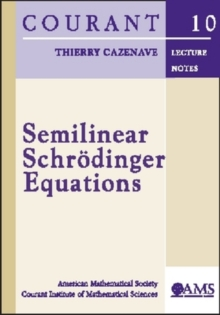Supporting your high street Find out how »
• My Account# Semilinear Schrodinger Equations Paperback / softback

## Part of the Courant Lecture Notes series

#### Description

The nonlinear Schrodinger equation has received a great deal of attention from mathematicians, particularly because of its applications to nonlinear optics.

It is also a good model dispersive equation, since it is often technically simpler than other dispersive equations, such as the wave or the Korteweg-de Vries equation.

From the mathematical point of view, Schrodinger's equation is a delicate problem, possessing a mixture of the properties of parabolic and elliptic equations.

Useful tools in studying the nonlinear Schrodinger equation are energy and Strichartz's estimates.This book presents various mathematical aspects of the nonlinear Schrodinger equation.

It studies both problems of local nature (local existence of solutions, uniqueness, regularity, smoothing effect) and problems of global nature (finite-time blowup, global existence, asymptotic behavior of solutions).

In principle, the methods presented apply to a large class of dispersive semilinear equations.

The first chapter recalls basic notions of functional analysis (Fourier transform, Sobolev spaces, etc).

Otherwise, the book is mostly self-contained. It is suitable for graduate students and research mathematicians interested in nonlinear partial differential equations and applications to mathematical physics.

#### Information

• Format: Paperback / softback
• Pages: 323 pages
• Publisher: American Mathematical Society
• Publication Date:
• Category: Mathematics
• ISBN: 9780821833995

£51.95

on all orders

###### Pick up orders

from local bookshops

£58.95

£58.85

£98.50

£97.39

£29.95

£29.39

£39.95